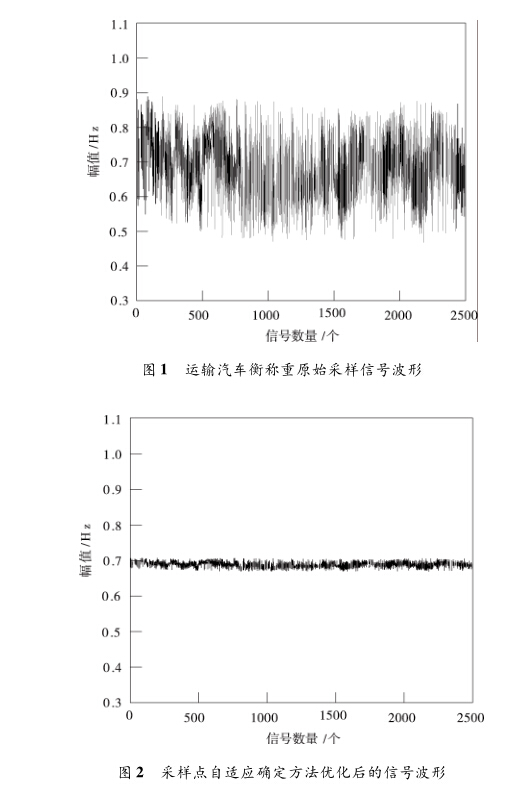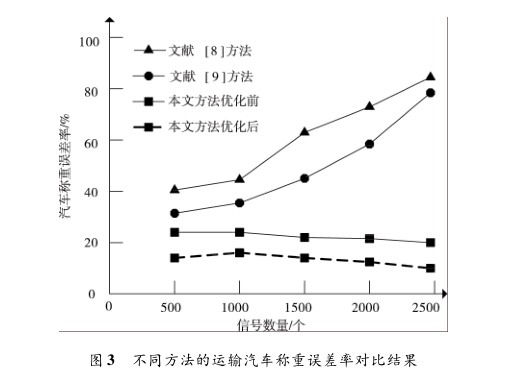# 运输地磅称重量精度优化仿真

1.引言

2.运输地磅称重量精度优化原理

MX + CX + KX = F (1)

X( t)

G( t)

F( t)

u(t)=[r(t) - r{t - £o)-

to — £"o) + T (t — to — 2so)]bo + 1 z  + b2 z  + 63 z 3 + 64 z 4 + 65z 5 + b6z 6

«1 g(n - 1) + a2g(n -2) + 03g(n -2) + 04g(n -2)

+ 05g (n - 2) + a6g (n  2) = 6x (n) + 6

x (n) +

1 x (n) + 62 x (n) + 63 x (n) + 64 x (n) + 65 x (n)

+ 66x (n) (5)

g(n -2) = g(n - 3) = g(n -4)

=g(n -5) = g(n -6) = g(n) (6)

g (n) = 6'ov (n) + 6'1 v (n - 1) + 6'2v (n - 2) + 6'3v (n -3)

+ 6'4v (n-4) + 6'5v (n-5) + 6'6v (n-6) + w (n) (7)

3.运输地磅称重量精度优化方法

3.1基于采样点自适应确定的信号采集和分析

y( t') =m (t ) + n (t ) (8)

7J = A1A2 …冷 1o)

= rot (z',) trans (z', ) trans (x, a;) rot (x, a) rot (y,,;) (11)

Ap = J- Ap (13)

Ap = (JTJ) 1JT Ap (14)

1 N( h)

r(h) = 2^g [ Ap(e{i')) - Ap(e{i') +h) ]2 (15)

a p^ =乏一')Af (16) i = 1

3.2基于采样点自适应确定的信号处理

,= X:i (17)

n i = 1

vi = i -X (18)

e = lin /— g (:i -x)2 (19) 一槡 n

I = \xb -X I > 3 (20)

3.3运输地磅称重采样点自适应确定方法优化

1采样点个数最少；

2最优采样点能够使得运输汽车所有目标点在精度补 偿后的剩余误差之和最小；

3最优采样点需要在指定的工作范围内选择；

4最优采样点需要使得运输汽车衡称重时的各个目标 点在精度补偿后的剩余误差在给定的精度要求区间内。

min/1 = Q (21)

L

minf2 = g I Ap, - Ap^ 1 (22)

i'=1

1  e(i') ru

s. t. { u (23)

11 a, - ApUi') 1  s

^i = DkUk A77^qt (24)

4.仿真结果与分析5.结束语